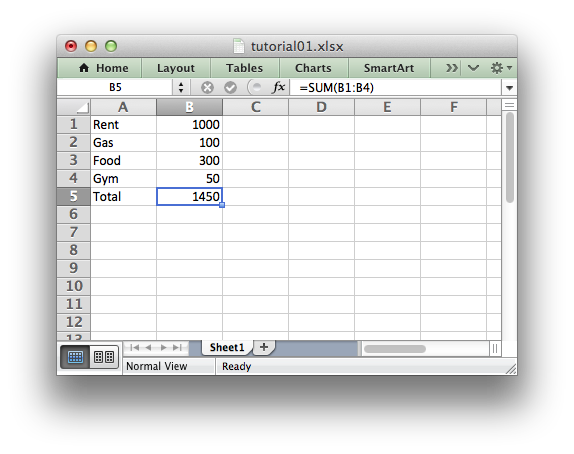# Tutorial 1: Create a simple XLSX file

Let’s start by creating a simple spreadsheet using Python and the XlsxWriter module.

Say that we have some data on monthly outgoings that we want to convert into an Excel XLSX file:

```expenses = (
['Rent', 1000],
['Gas',   100],
['Food',  300],
['Gym',    50],
)
```

To do that we can start with a small program like the following:

```import xlsxwriter

# Create a workbook and add a worksheet.
workbook = xlsxwriter.Workbook('Expenses01.xlsx')

# Some data we want to write to the worksheet.
expenses = (
['Rent', 1000],
['Gas',   100],
['Food',  300],
['Gym',    50],
)

# Start from the first cell. Rows and columns are zero indexed.
row = 0
col = 0

# Iterate over the data and write it out row by row.
for item, cost in (expenses):
worksheet.write(row, col,     item)
worksheet.write(row, col + 1, cost)
row += 1

# Write a total using a formula.
worksheet.write(row, 0, 'Total')
worksheet.write(row, 1, '=SUM(B1:B4)')

workbook.close()
```

If we run this program we should get a spreadsheet that looks like this:This is a simple example but the steps involved are representative of all programs that use XlsxWriter, so let’s break it down into separate parts.

The first step is to import the module:

```import xlsxwriter
```

The next step is to create a new workbook object using the `Workbook()` constructor.

`Workbook()` takes one, non-optional, argument which is the filename that we want to create:

```workbook = xlsxwriter.Workbook('Expenses01.xlsx')
```

Note

XlsxWriter can only create new files. It cannot read or modify existing files.

The workbook object is then used to add a new worksheet via the `add_worksheet()` method:

```worksheet = workbook.add_worksheet()
```

By default worksheet names in the spreadsheet will be Sheet1, Sheet2 etc., but we can also specify a name:

```worksheet1 = workbook.add_worksheet()        # Defaults to Sheet1.
worksheet3 = workbook.add_worksheet()        # Defaults to Sheet3.
```

We can then use the worksheet object to write data via the `write()` method:

```worksheet.write(row, col, some_data)
```

Note

Throughout XlsxWriter, rows and columns are zero indexed. The first cell in a worksheet, `A1`, is `(0, 0)`.

So in our example we iterate over our data and write it out as follows:

```# Iterate over the data and write it out row by row.
for item, cost in (expenses):
worksheet.write(row, col,     item)
worksheet.write(row, col + 1, cost)
row += 1
```

We then add a formula to calculate the total of the items in the second column:

```worksheet.write(row, 1, '=SUM(B1:B4)')
```

Finally, we close the Excel file via the `close()` method:

```workbook.close()
```

And that’s it. We now have a file that can be read by Excel and other spreadsheet applications.

In the next sections we will see how we can use the XlsxWriter module to add formatting and other Excel features.